Search IntMath
Close

450+ Math Lessons written by Math Professors and Teachers

5 Million+ Students Helped Each Year

1200+ Articles Written by Math Educators and Enthusiasts

Simplifying and Teaching Math for Over 23 Years

# Hubble math

By Murray Bourne, 15 Jun 2009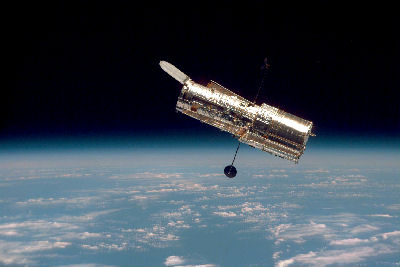The Hubble Telescope [Source]

I came across this PowerPoint presentation about Hubble recently. The opening statement has a few errors. It says:

The Hubble Space Telescope is a robotic telescope located on the outer edge of the atmosphere, in circular orbit around the Earth at 593 km above sea level, with an orbital period between 96 and 97 minutes at a speed [of] 28,000 km/h. Named as a tribute to Edwin Hubble, [it] was launched into orbit on 24 April 1990 as a joint project of NASA and ESA, thus inaugurating the Great Observatories program. [The] cylindrically shaped Hubble weighs 11 tons. Its length is 13.2 m and its maximum diameter is 4.2 meters. The telescope can obtain images with optical resolution [finer than] 0.1 seconds of arc.

The first thing that struck me was the "circular orbit". All satellites, whether the moon around us, or the Earth around the sun, (or any satellite we have sent into space) orbits along an elliptical path. (An ellipse is a stretched circle.) This was discovered by Kepler in the 17th century and stated in his First Law of Planetary Motion:

The orbit of every planet is an ellipse with the sun at a focus.

To be fair, though, many orbits are very nearly circular. The amount of stretch of an ellipse is known as its eccentricity, [See more on ellipses.]

The following image clearly shows how low the orbit is. In fact, the Earth is "in the way" about half the time.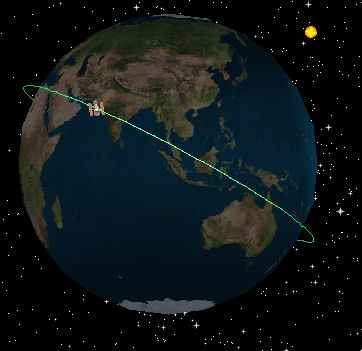Low Earth Orbit [Image from the European Space Agency applet where you can see where the Hubble is right now.]

## Weight of the Hubble

The Hubble does not weigh 11 tons right now. It is weightless. You only experience "weight" when you are being affected by gravity. While the Earth's gravity still acts on the Hubble, like all orbiting bodies it experiences "free fall", so is weightless.

More accurately, Hubble's mass is 11 tonnes. It would weigh about 108,000 N on the Earth.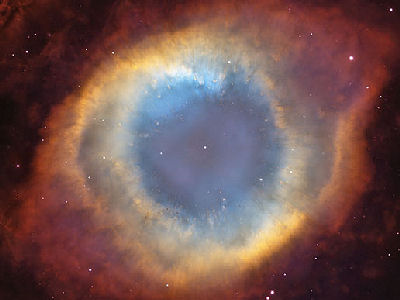Helix Nebula [Image source]

## Optical resolution - seconds of arc

"The telescope can obtain images with optical resolution [greater than] 0.1 seconds of arc."

This is actually quite interesting. What does "0.1 seconds of arc" mean?

Angles are commonly measured in degrees. We know that a right angle is 90° and that a steep slope for a road is 15°. One complete revolution is 360°.

Here is a small angle (the smallest I can show without it looking like a single line), 2°, also called "2° of arc".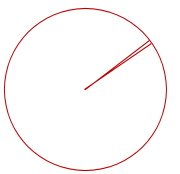The Hubble Space Telescope needs to "see" very small parts of the sky. When we want smaller units than one degree, we take divisions of 1/60 of a degree, called minutes (just like 1/60th of an hour is one minute. Such divisions come from the ancient Babylonians).

If minutes are not small enough, we take 1/60th of a minute, to give us seconds (just like in units of time).

So "one second of arc" means 1/3600th of one degree.

But Hubble can do even better than that - it can discern a field of view which is 1/10 of 1/3600th, or 1/36,000th of one degree. Not bad!

In science and engineering, the radian is a more convenient unit for measuring angles than the degree. One radian is the angle shown in this diagram. If we rotate around a circle such that we travel the distance of one radius, we will have traced out an angle of one radian at the center. It's about 57.3°.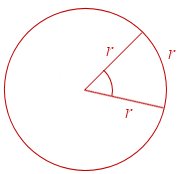So in radians, the Hubble can distinguish around 4.8 × 10-7 of arc.

To finish, here's one of my favorite pictures by Hubble. It's a star that erupted in Jan 2002, and it was 600,000 times more luminous than our Sun.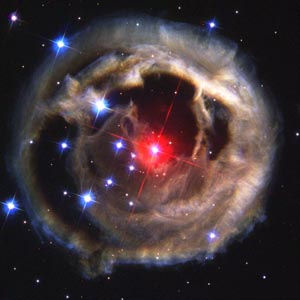[Image source: NASA]

Of course, the star erupted 20,000 years ago and it took that long for the light to reach us.

### 6 Comments on “Hubble math”

1. Anthony says:

If you're going to be a pedant about the weight/mass thing, at least don't introduce even larger errors by yourself.

> More accurately, Hubble’s mass is 11 tonnes. It would weigh about 108 N on the Earth.

108,000N is the figure you're looking for.

2. Murray says:

Oops - thanks, Anthony! I have updated the post.

3. Steven says:

I don't think Zac's a "pedant" for pointing out something which is basic physics.

There is a difference between mass and weight.

4. David says:

I am a fan of the HST. I have many, many images taken by Hubble. I have always been curious about one thing though. Why are Hubble's images not rectangular, sort of in the shape of the B2 Stealth Bomber?
Thank you,
Dave

5. fatcat says:

Actually the HST does have weight. It's weight is its mass times the gravity it experiences (around 8.2 m s^-2).
You can do the maths.
Yes it is 'weightless' but this is a term to describe the experience of being in free fall, it does not mean having no weight.

6. Hubble Space Telescope: the source of amazing pictures and science - Asbronomers says:

[…] HST’s orbit around Earth. Source: ESA applet, found with https://www.intmath.com/blog/mathematics/hubble-math-2411 […]

### Comment Preview

HTML: You can use simple tags like <b>, <a href="...">, etc.

To enter math, you can can either:

1. Use simple calculator-like input in the following format (surround your math in backticks, or qq on tablet or phone):
a^2 = sqrt(b^2 + c^2)
(See more on ASCIIMath syntax); or
2. Use simple LaTeX in the following format. Surround your math with $$ and $$.
$$\int g dx = \sqrt{\frac{a}{b}}$$
(This is standard simple LaTeX.)

NOTE: You can mix both types of math entry in your comment.